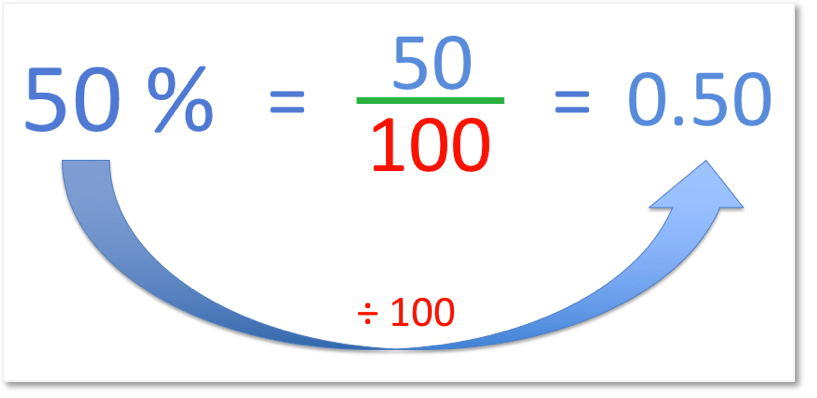# Convert Fractions to Percentages without a Calculator

Convert Fractions to Percentages without a Calculator• The word per means ‘out of’ and cent means ‘100’.
• Percent means ‘out of 100’.
• We can take the of a fraction out of 100 and write it as a percentage.
• We basically replace the   / 100   with a percentage sign, %.
• The percentage sign, % is an easier way to write ‘out of 100’.
• In this example   73 / 100 is written as 73%.
• We read this as 73 percent.

Percent means ‘out of 100’.

We can take a fraction out of 100 and write its numerator as a percentage.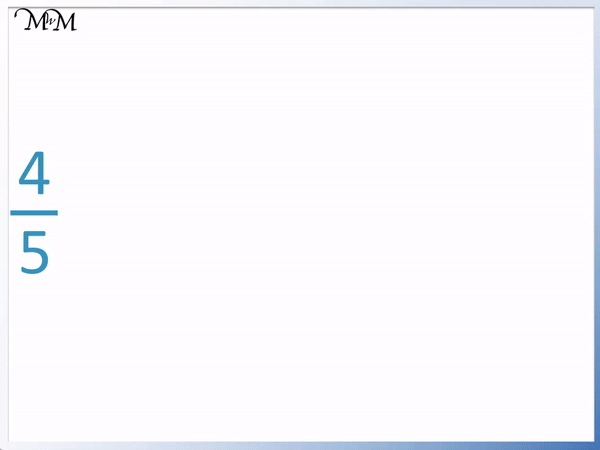• To write a fraction as a percentage we first need to write it as a fraction out of 100.
• We write a fraction out of 100 by using equivalent fractions.
• This means that we must multiply the and the by the same amount.
• We can first multiply the top and bottom of the fraction   4 / 5   by 2.
• After multiplying the top and bottom by 2, we get   8 / 10  .
• We can now write   8 / 10   as   80 / 100   by multiplying the top and bottom both by 10.
• We could have written   4 / 5   as   80 / 100   in one go by multiplying the top and bottom by 20.
•   4 / 5   is 80 out of 100, which we can write as 80%.# Converting Fractions to Percentages without a Calculator

## What is a Percentage?

A percentage is a value out of 100. Percent means ‘out of 100’.

We can write the number as a fraction out of 100 or we can use the % symbol. The % symbol is a short way to write ‘out of 100’ as a fraction.

The word ‘percent’ is made up of two parts.

The ‘per’ means ‘out of’ and the ‘cent’ means ‘100’.

In our minds, we can replace the word ‘percent’ with the words ‘out of 100’.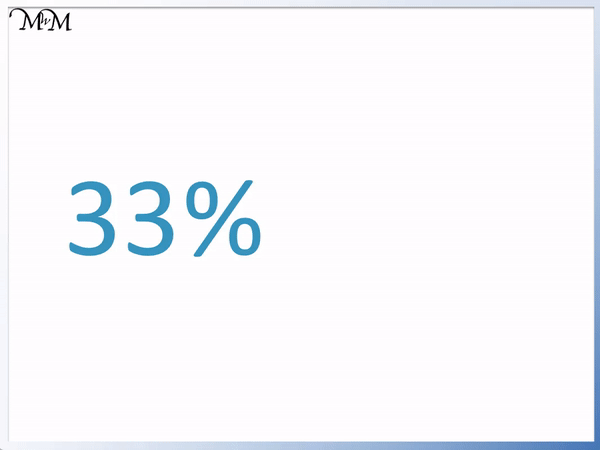For example, 33% just means 33 out of 100. This can also be written as a fraction as   33 / 100  .

Here is 73 percent.

73 percent is 73 out of 100.We can write this as   73 / 100  .

The percent symbol, ‘%’ is often used instead of writing   / 100  .

We can write 73 out of 100 as 73%. The % sign can be thought of as meaning ‘out of 100’.In this example, we have 2 percent.

We can replace the word ‘percent’ with the words ‘out of 100’.

2 percent means 2 out of 100.We can write this as   2 / 100  .

We can write this more easily as 2%.## How to Convert a Fraction to a Percentage without a Calculator

To convert fractions to percentages without a calculator, use the following steps:

• Multiply the denominator (bottom) of the fraction by a number to make the denominator equal 100.
• Multiply the numerator (top) of the fraction by the same number.
• Take this new numerator and write a % sign after it.

If the fraction is already out of 100, then we simply write the numerator with a percentage sign after it.

For example, the fraction of   12 / 100   is already out of 100.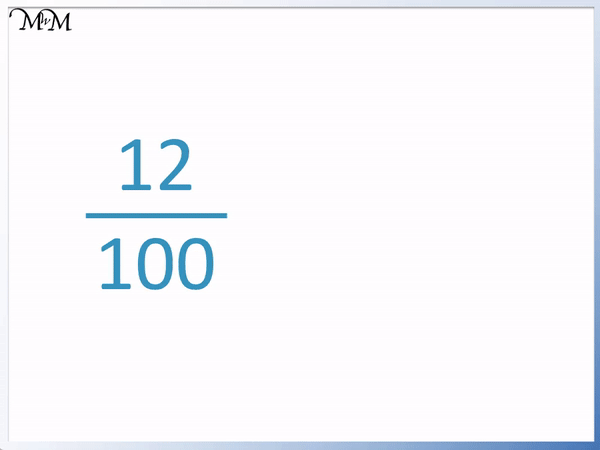We simply take the numerator on top of the fraction, which is 12, and write a percentage sign after it.

12 / 100   is 12%.

We will now look at examples of writing a fraction as a percentage without a calculator, where the fraction is not out of 100.

Here we have the fraction   8 / 50  .

We can see that it is out of 50, not out of 100.

The first step is to write it as an equivalent fraction out of 100.We need to multiply the 50 to make it 100. We need to figure out how many times 50 divides into 100.

50 × 2 = 100 and so, we multiply the denominator by 2.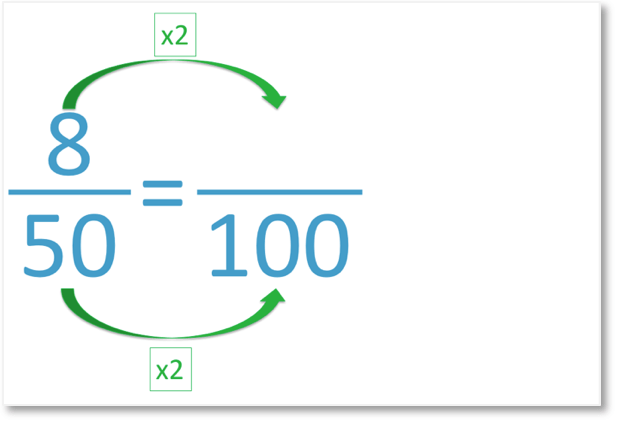When finding equivalent fractions, we need to multiply the numbers on top and bottom by the same amount.

Because we multiplied 50 by 2, we need to multiply 8 by 2 as well.

8 × 2 = 16 and so our fraction is now   16 / 100  .8 / 50   is exactly the same amount as   16 / 100  . We say that these fractions are equivalent.Now that our fraction is our of 100, it is a percentage.

A percentage is just a fraction out of 100.

We can write   16 / 100   more easily at 16%.

We take the numerator of 16 and put a percentage sign after it.

The fraction   8 / 50   written as a percentage is 16%.

This also means that 8 is 16% of 50.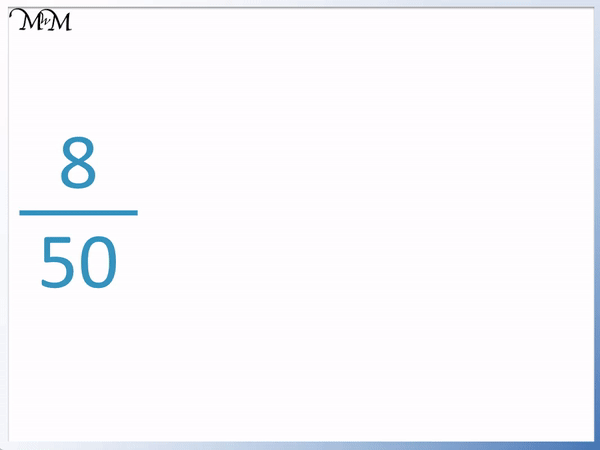Here is another example of writing 11 out of 25 as a percentage without a calculator.

The first step is to multiply the number on the bottom so that it equals 100. Remember that we need our fraction to be out of 100, not 25.

25 goes into 100 four times and so, we multiply by 4.The next step is to multiply the number on the top of the fraction by the same amount. Because we multiplied the bottom by 4, we will multiply the top by 4 too.The fraction   11 / 25   is equivalent to   44 / 100  .

11 / 25   is 44%.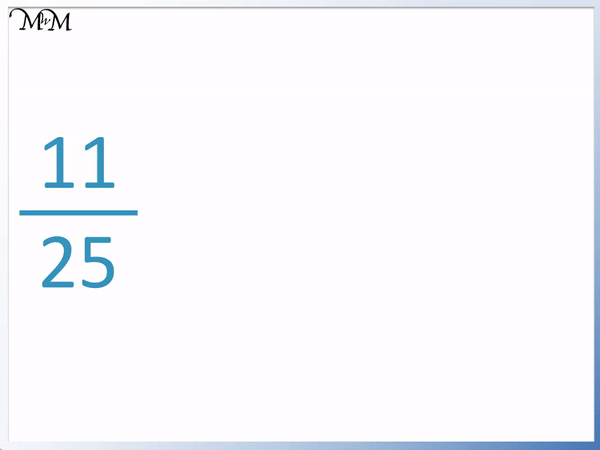In this example we have   7 / 20  .

20 goes into 100 five times and so, we multiply the top and bottom of the fraction by 5.Multiplying the numerator by 5, we get 35.

7 / 20   is the same as   35 / 100  .The fraction   7 / 20   is 35%.

This also means that 7 is 35% of 20.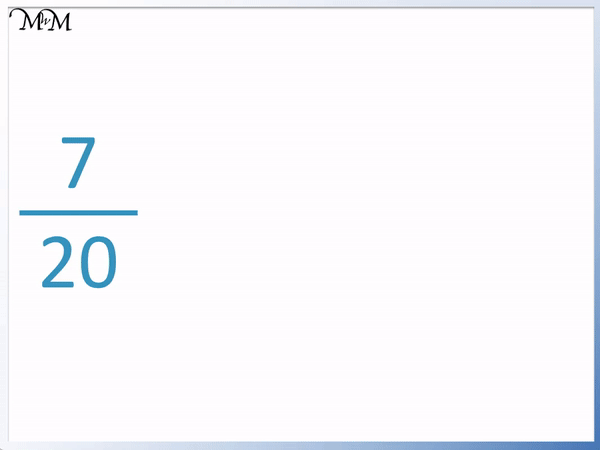In this example of turning fractions into percentages, we have   3 / 10  .We multiply the numerator and the denominator both by 10 to find an equivalent fraction out of 100.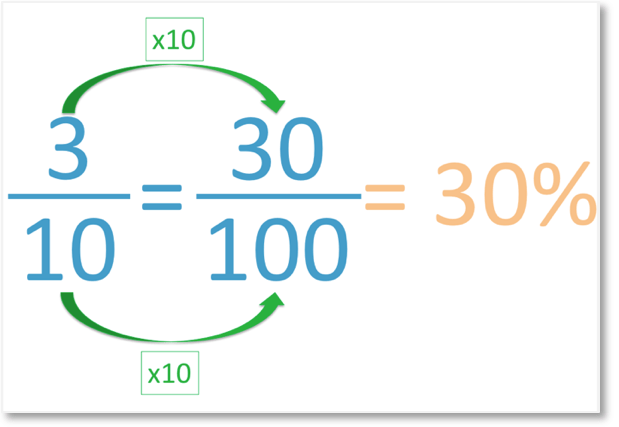3 out of 10 is 30 out of 100.

3 / 10   is 30%.This also means that 3 is 30% of 10.

So far we have been able to convert fractions into percentages without a calculator because we could use our times tables to make fractions out of 100.

Sometimes it is not so obvious how to make a fraction out of 100. In this case, we can multiply in steps.

Here is   4 / 5  . The first step to turn this fraction into a percentage is to make the number on the bottom equal to 100 by multiplying it.

You may not know how many times 5 goes into 100 and so, we can just make it equal 10 for now.

We can multiply the top and bottom by 2.We multiply 5 by 2 and so we multiply 4 by 2.

4 / 5   is equivalent to   8 / 10  .

We can now multiply 10 by 10 to make it equal 100.4 / 5   =   8 / 10   =   80 / 100  .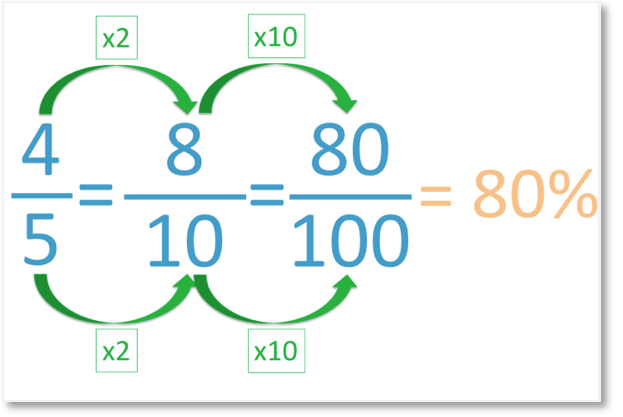80 / 100   is 80%.

So   4 / 5   is 80%.This also means that 4 is 80% of 5.

We can see that if we did this in one step, we still get the same answer.

We turn this fraction into a percentage without a calculator in one step. 100 ÷ 5 = 20 because 10 ÷ 5 = 2.We can multiply 4 by 20 and 5 by 20 to find our equivalent fraction in one step.

We still get 80 out of 100.80 out of 100 is 80%.Now try our lesson on Converting Percentages to Decimals where we learn how to write a percentage as a decimal.